# please solve this one calculus 2 QUESTION 4 Evaluate the integral by using a substitution prior...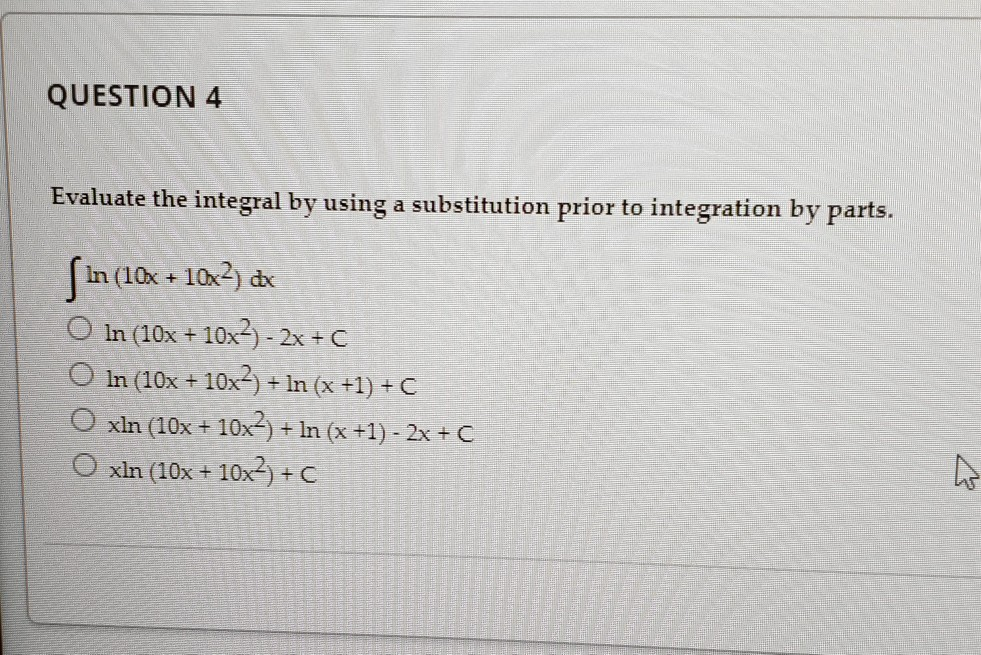calculus 2

QUESTION 4 Evaluate the integral by using a substitution prior to integration by parts. ſin (10x + 10x2) de O in (10x + 10x2) - 2x + c O ln (10x + 10x>) + ln (x+1)+C O xin (10x + 10x2 + In (x +1) - 2x +C O xln (10x + 10x2)+C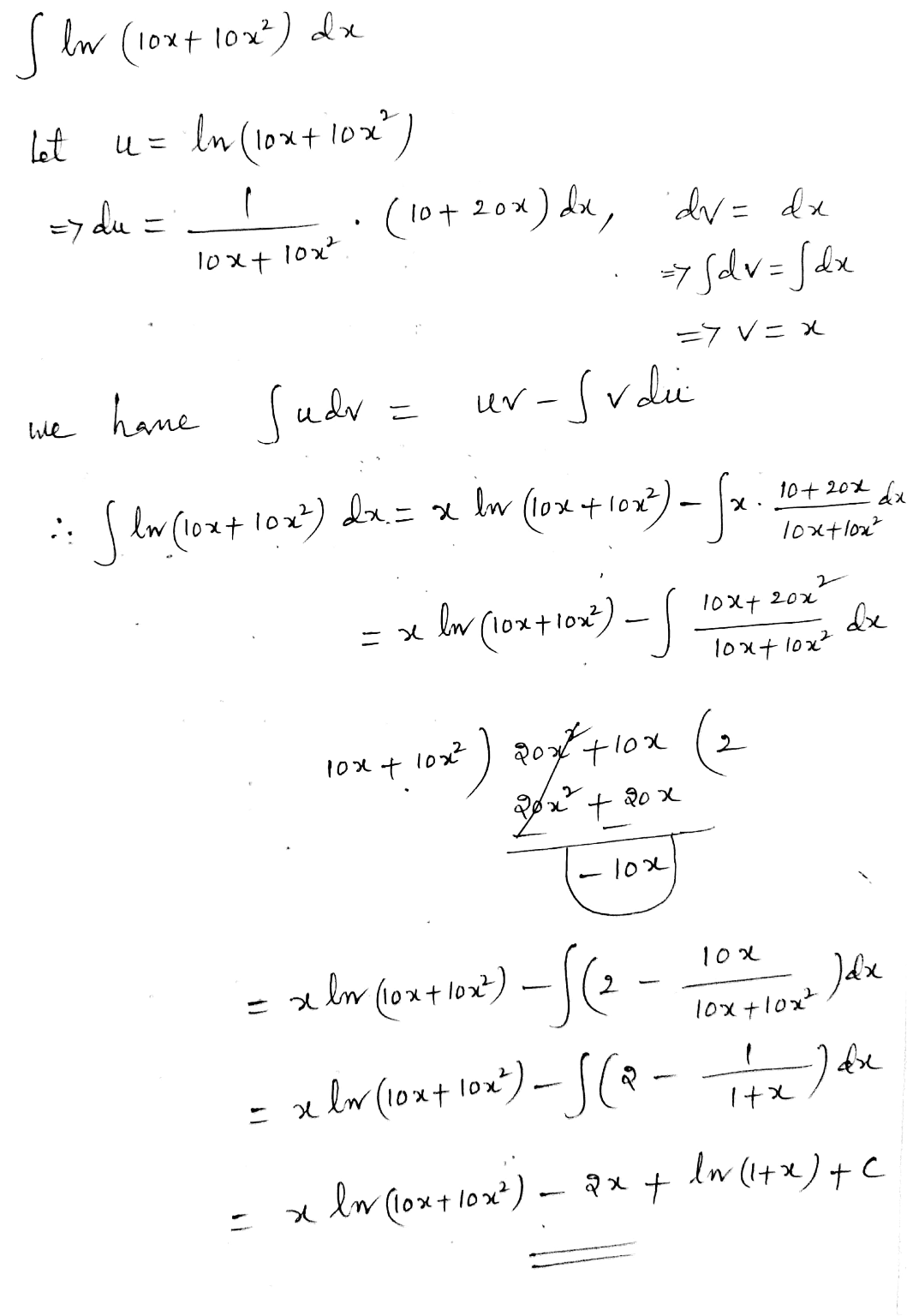#### Earn Coin

Coins can be redeemed for fabulous gifts.

Similar Homework Help Questions
• ### Evaluate the following integral. This involves substitution and integration of ln. \int 3 2 (x ^4...

Evaluate the following integral. This involves substitution and integration of ln. \int 3 2 (x ^4 + 1 )/(x ^5 + 5x )dx

• ### 2. (20 points) Evaluate the following integral using Integration by Parts or Trigonometric Substitution 4.r3 dc...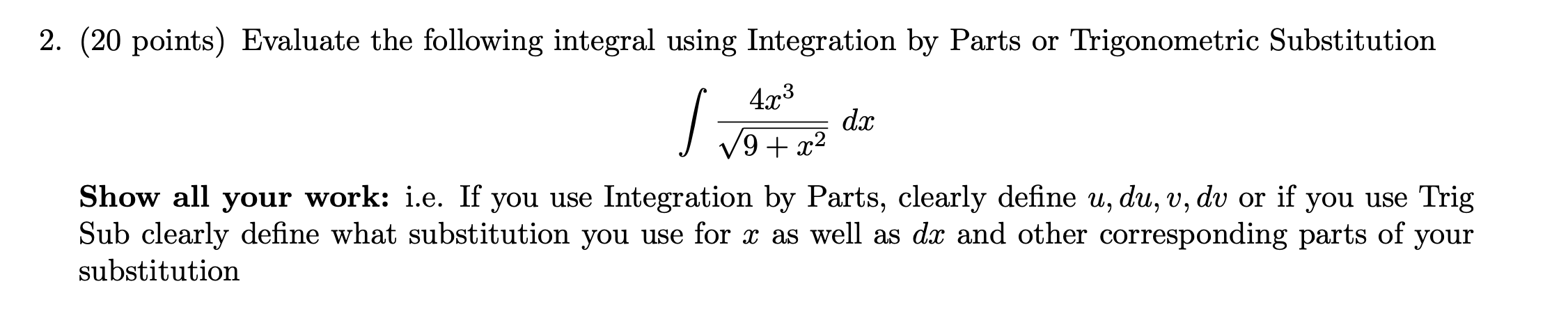2. (20 points) Evaluate the following integral using Integration by Parts or Trigonometric Substitution 4.r3 dc 9 +22 4 Show all your work: i.e. If you use Integration by Parts, clearly define u, du, v, dv or if you use Trig Sub clearly define what substitution you use for x as well as dx and other corresponding parts of your substitution

• ### please help me with the question in the photo 2. (20 points) Evaluate the following integral...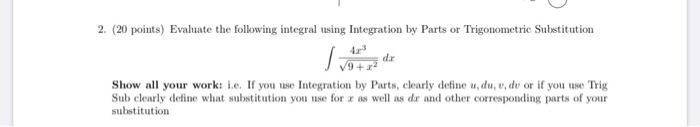please help me with the question in the photo 2. (20 points) Evaluate the following integral using Integration by Parts or Trigonometric Substitution Show all your work: 1.c. If you use Integration by Parts, clearly define udu, v, du or if you use Trig Sub clearly define what substitution you use for r as well as dx and other corresponding parts of your substitution

• ### help please Evaluate the definite integral using the Fundamental Theorem of Calculus. (1+ (1 + 14х5)...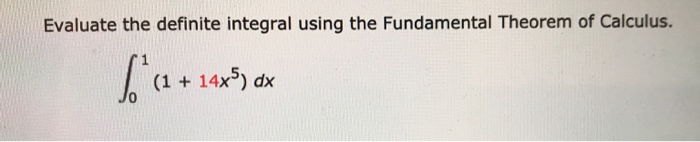help please Evaluate the definite integral using the Fundamental Theorem of Calculus. (1+ (1 + 14х5) dx Use The Fundamental Theorem of Calculus and the antiderivative found in Step 2 to evaluate the definite integral. fo* (2 + 14x5) dx = = (x+3x0916 (1+](O* )-( O*+O) “) 10 3

• ### 3. State whether the given integrals should be solved using Integration by Parts or u-Substitution. but...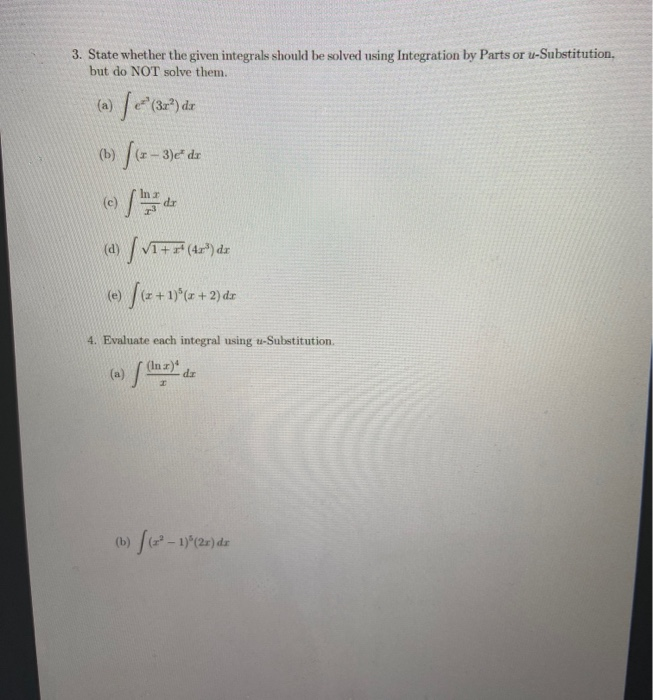3. State whether the given integrals should be solved using Integration by Parts or u-Substitution. but do NOT solve them. /2"(34) de (b) / (+ 3)e+ dr (c) (a) / 1+ ** (42") der (2+1)* (x + 1)(x + 2) dr (e) 4. Evaluate each integral using t-Substitution da (b) / (+" - 13% (26) ds

• ### 2. (20 points) Evaluate the following integral using Integration by Parts or Trigonometric Substitution dar V9...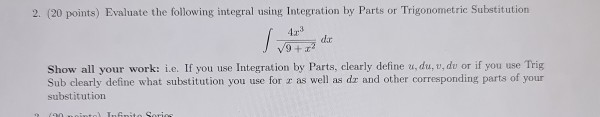2. (20 points) Evaluate the following integral using Integration by Parts or Trigonometric Substitution dar V9 +2 Show all your work: i.e. If you use Integration by Parts, clearly define u du, du or if you use Trig Sub clearly define what substitution you use for x as well as dy and other corresponding parts of your substitution

• ### (a) Use Trigonometric Substitution to evaluate the integral 22 9 dr. T (b) Use the method...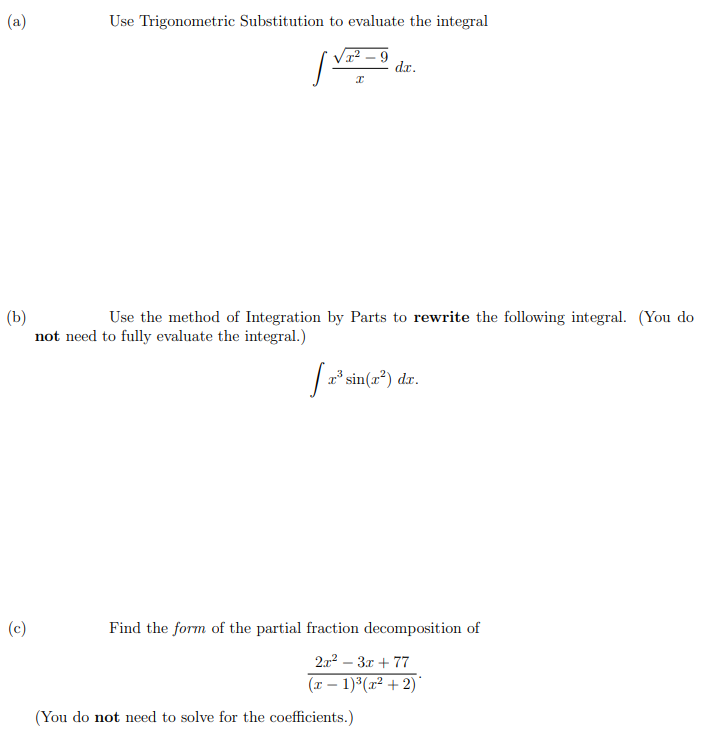(a) Use Trigonometric Substitution to evaluate the integral 22 9 dr. T (b) Use the method of Integration by Parts to rewrite the following integral. (You do not need to fully evaluate the integral.) | «* sin(x2) dr. (c) Find the form of the partial fraction decomposition of 2.r2 - 3.c + 77 (x - 1)(x² +2) (You do not need to solve for the coefficients.)

• ### 3. Evaluate the definite integral using substitution. (10 points) 2-1 de so xede 4. Find the...3. Evaluate the definite integral using substitution. (10 points) 2-1 de so xede 4. Find the equation of the tangent line to the graph of the function f(x) at the given point. (10 points) f(x) = 7+2-2 (1,1) 5. Evaluate the definite integral using substitution. (10 points) ""(tant + 1)* see t dit

• ### 2. (20 points) Evaluate the following integral using Integration by Parts or Trigonometric Substitution dr Show...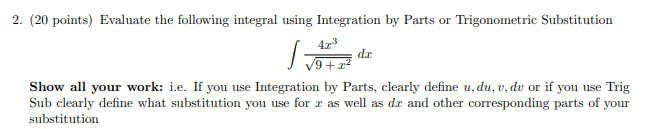2. (20 points) Evaluate the following integral using Integration by Parts or Trigonometric Substitution dr Show all your work: i.e. If you use Integration by Parts, clearly define u,du, v, dv or if you use Trig Sub clearly define what substitution you use for r as well as dr and other corresponding parts of your substitution

• ### solve 1 and 2. Evaluate the integral. 3T/4 1) rt/4 D) o B)-16 C) Find the derivative of the integral using the Seco...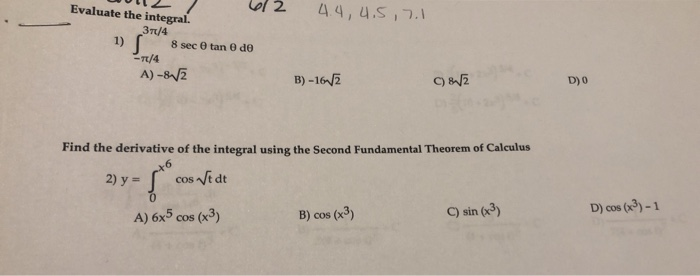solve 1 and 2. Evaluate the integral. 3T/4 1) rt/4 D) o B)-16 C) Find the derivative of the integral using the Second Fundamental Theorem of Calculus 2) y- cos nt dt D) cos (3)-1 C) sin (3) B) cos (x3) A) 6x5 cos (x3) Evaluate the integral. 3T/4 1) rt/4 D) o B)-16 C) Find the derivative of the integral using the Second Fundamental Theorem of Calculus 2) y- cos nt dt D) cos (3)-1 C) sin (3) B)...